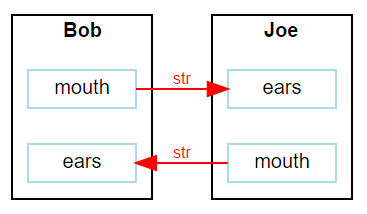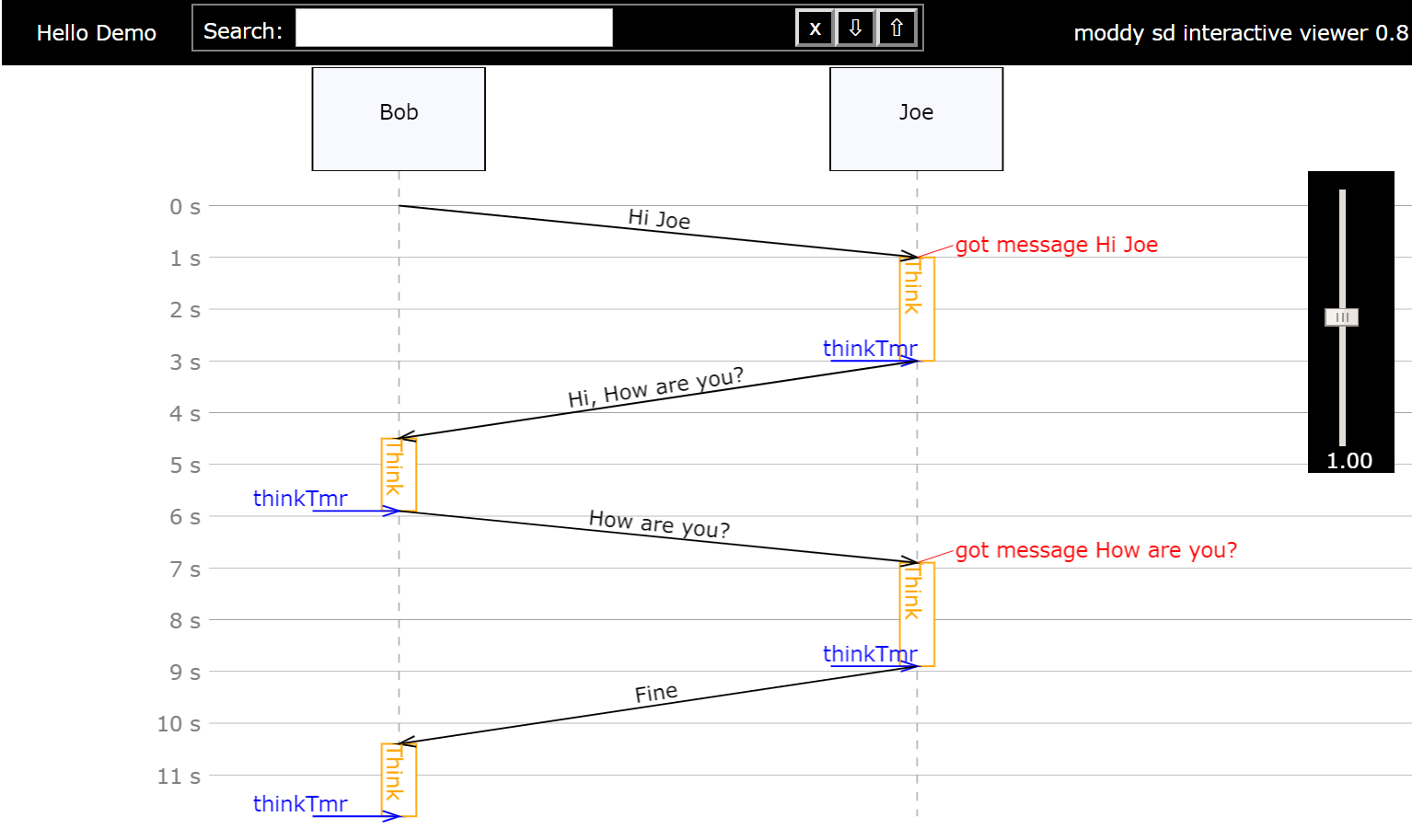# Quick Start¶

The following chapter describes the basic functions of Moddy by using the example Hello tutorial model.

In a Moddy model, a system is composed of parts that communicate with other parts through messages. In the Hello tutorial, we simulate a conversation between two people, “Bob” and “Joe”, therefore “Bob” and “Joe” are the two parts used in the model.

Note

This example uses only only of two modelling flavours, the “event based” method. Using this method, the model reacts to events using callback routines.

Another modelling technique is the “program based” method. See Hello Demo as a Sequential Program as an example and Sequential Program Simulation for more information.

## Model Structure¶

The structure of the system is modelled via parts, ports and bindings between ports. In the Hello tutorial, we have two parts, Bob and Joe. Each part has an output port (mouth) and an input port (ears).

To simulate the time that Bob and Joe need for thinking, we create also a timer for both. To model the behavior of Bob, we define a class “Bob”, which creates the ports and timers:

```    import moddy

class Bob(moddy.SimPart):
''' Model of Bob '''
def __init__(self, sim, obj_name):
# Initialize the parent class
super().__init__(sim=sim, obj_name=obj_name,
elems={'in': 'ears',
'out': 'mouth',
'tmr': 'think_tmr'})
...
```

The main program creates an instance of Bob and Joe like this

```SIMU = moddy.Sim()

Bob(SIMU, "Bob")
Joe(SIMU, "Joe")
```

To allow Joe to hear what Bob says, we “bind” the ears of Joe to the mouth of Bob and vice versa.

```SIMU.smart_bind([['Bob.mouth', 'Joe.ears'], ['Bob.ears', 'Joe.mouth']])
```

Moddy can also output the structure of the model. After you created the structure, call `gen_dot_structure_graph()`:

```# Output model structure graph
moddy.gen_dot_structure_graph(SIMU, 'output/1_hello_structure.svg')
```

This is the resulting structure graph:## Model Behavior¶

To model the behavior of parts, an event based model:

• Sends messages to other parts

• Reacts on received messages from other parts

• Reacts on timer events

This means, the simulator calls the model code only whenever such an event occurs. For example, Bob’s behavior will be modelled as follows:

```...
def ears_recv(self, _, msg):
''' Callback for message reception on ears port '''
if msg == "Hi, How are you?":
else:

self.think_tmr.start(1.4)
self.set_state_indicator("Think")

def think_tmr_expired(self, _):
''' Callback for think_tmr expiration '''
self.set_state_indicator("")
# pylint: disable=no-member
```

## Ports and Messages¶

A message is send always from an “Output Port” to an “Input Port”.

A part can have many Ports to communicate with other parts.

A message is send via the sending port’s `send()` method:

```self.mouth.send(self.reply, 1)
```

In this example, self.reply is the message; here it is a string. The second parameter defines the flight time, i.e. how long it takes until the message arrives at the input port. On the receiver side, the part that owns the input port must define a “receive function”, which gets passed the message just received:

```def ears_recv(self, _, msg):
''' Callback for message reception on ears port '''
if msg == "Hi, How are you?":
else:

self.think_tmr.start(1.4)
self.set_state_indicator("Think")
```

Note

This receive routine must be called always <portName>_recv.

## Timers¶

A part can have many timers to control its own behavior. A timer is stopped by default.

```def think_tmr_expired(self, _):
''' Callback for think_tmr expiration '''
self.set_state_indicator("")
```

Note

The expiration routine must be called always <timerName>_expired.

## Running Simulator¶

After the parts and bindings were created, the simulator can `run()`

```# let simulator run
SIMU.run(stop_time=12.0)
```

Here we stop the simulator after 12 seconds. If no limit is given, the simulator would run until no more events to execute. On the python console, the simulator outputs the simulation trace:

```TRC:       0.0s >MSG    Bob.mouth(OutPort) //  req=0.0s beg=0.0s end=1.0s dur=1.0s msg=[Hi Joe]
SIM: Simulator 1.6.1 starting
TRC:       1.0s <MSG    Joe.ears(InPort) //  req=0.0s beg=0.0s end=1.0s dur=1.0s msg=[Hi Joe]
TRC:       1.0s ANN     Joe(Part) // got message Hi Joe
TRC:       1.0s T-START Joe.thinkTmr(Timer) // 2.0s
TRC:       1.0s STA     Joe(Part) // Think
TRC:       3.0s T-EXP   Joe.thinkTmr(Timer)
TRC:       3.0s STA     Joe(Part) //
TRC:       3.0s >MSG    Joe.mouth(OutPort) //  req=3.0s beg=3.0s end=4.5s dur=1.5s msg=[Hi, How are you?]
TRC:       4.5s <MSG    Bob.ears(InPort) //  req=3.0s beg=3.0s end=4.5s dur=1.5s msg=[Hi, How are you?]
TRC:       4.5s T-START Bob.thinkTmr(Timer) // 1.4s
TRC:       4.5s STA     Bob(Part) // Think
TRC:       5.9s T-EXP   Bob.thinkTmr(Timer)
TRC:       5.9s STA     Bob(Part) //
TRC:       5.9s >MSG    Bob.mouth(OutPort) //  req=5.9s beg=5.9s end=6.9s dur=1.0s msg=[How are you?]
TRC:       6.9s <MSG    Joe.ears(InPort) //  req=5.9s beg=5.9s end=6.9s dur=1.0s msg=[How are you?]
TRC:       6.9s ANN     Joe(Part) // got message How are you?
TRC:       6.9s T-START Joe.thinkTmr(Timer) // 2.0s
TRC:       6.9s STA     Joe(Part) // Think
TRC:       8.9s T-EXP   Joe.thinkTmr(Timer)
TRC:       8.9s STA     Joe(Part) //
TRC:       8.9s >MSG    Joe.mouth(OutPort) //  req=8.9s beg=8.9s end=10.4s dur=1.5s msg=[Fine]
TRC:      10.4s <MSG    Bob.ears(InPort) //  req=8.9s beg=8.9s end=10.4s dur=1.5s msg=[Fine]
TRC:      10.4s T-START Bob.thinkTmr(Timer) // 1.4s
TRC:      10.4s STA     Bob(Part) // Think
TRC:      11.8s T-EXP   Bob.thinkTmr(Timer)
TRC:      11.8s STA     Bob(Part) //
TRC:      11.8s >MSG    Bob.mouth(OutPort) //  req=11.8s beg=11.8s end=12.8s dur=1.0s msg=[Hm?]
SIM: Stops because stop_time reached
SIM: Simulator stopped at 12.0s. Executed 9 events in 0.001 seconds
saved sequence diagram in output/1_hello.html as iaViewer
Saved structure graph to output/1_hello_structure.svg
saved output/1_hello.csv as CSV
```

## Generating the Sequence Diagram¶

Moddy can generate a sequence diagram from the simulation results. The sequence diagram is generated as a as HTML. After simulation run, the following code generates the sequence diagram. For details, see `gen_interactive_seq_diagram()`:

```moddy.gen_interactive_sequence_diagram(SIMU,
file_name="output/1_hello.html",
time_per_div=1.0,
pix_per_div=30,
title="Hello Demo")
```

This is the result:View as HTML: `1_hello`

Notes:

• The black arrows are messages

• The blue arrows are timer expiration events

• The orange boxes are visualized “states” or “activities” that were generated by the model via `set_state_indicator()`.

• The red messages are annotations that were generated by the model via `annotation()`